## 9.1 Vectors

A vector is a sequence of data elements of the same type.

 329 45 12 28

### 9.1.1 Creating a vector

• Values are assigned to a vector using the c function (c for combining elements). Open and close the round brackets, and place the different elements, separated by commas.
``vector1 <- c(329, 45, 12, 28)``

You can create an empty vector with:

``````# empty vector
vecempty <- vector()
# empty numerical vector with 5 elements
vecnumempty <- vector(mode="numeric", length=5)
# empty character vector with 8 elements
veccharempty <- vector(mode="character", length=8)``````
• Create a sequence of consecutive numbers:
``````vecnum <- 10:16
# shortcut for:
vecnum <- c(10, 11, 12, 13, 14, 15, 16)
# NOTES: both ends (10 and 16) are included``````
• Character vectors: each element is entered between (single or double) quotes.
 mRNA miRNA snoRNA lncRNA
``mynames <- c("mRNA", "miRNA", "snoRNA", "lncRNA")``

HANDS-ON

1. Create a vector (choose the name) that contains a sequence of number, from 3 to 8, both included.
2. Create vector myfruits as:
``myfruits <- c(apple, pear, orange)``

What is happening? Why? Can you modify the line above so it doesn’t throw an error?

### 9.1.2 Vector manipulation

• A vector can be named: each element of the vector can be assigned a name (number or character)
``````# our previous numerical vector
vector1 <- c(329, 45, 12, 28)
# assign names
names(vector1) <- c("mRNA", "miRNA", "snoRNA", "lncRNA")
# use an object which already contains a vector
mynames <- c("mRNA", "miRNA", "snoRNA", "lncRNA")
names(vector1) <- mynames``````
• Get the length (number of elements) of a vector
``length(vector1)``
``##  4``
• Extracting elements from vector a
• extract elements using their position (index) in the vector: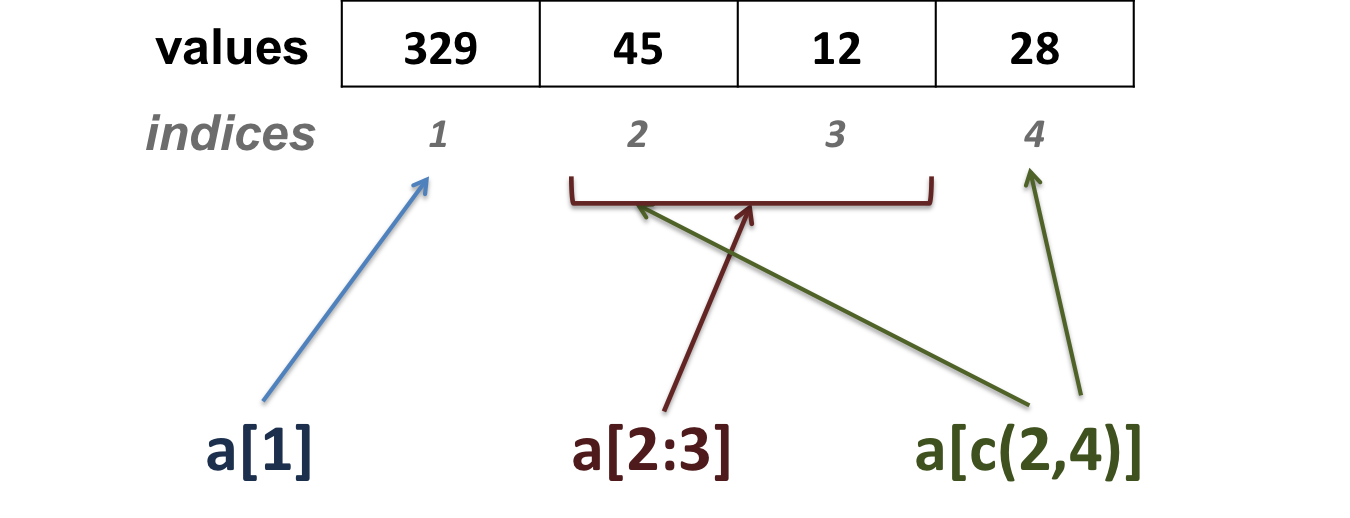``vector1``
``````## mRNA
##  329``````
``vector1[c(1,3)]``
``````##   mRNA snoRNA
##    329     12``````
``vector1[2:4]``
``````##  miRNA snoRNA lncRNA
##     45     12     28``````
• extract elements using their names: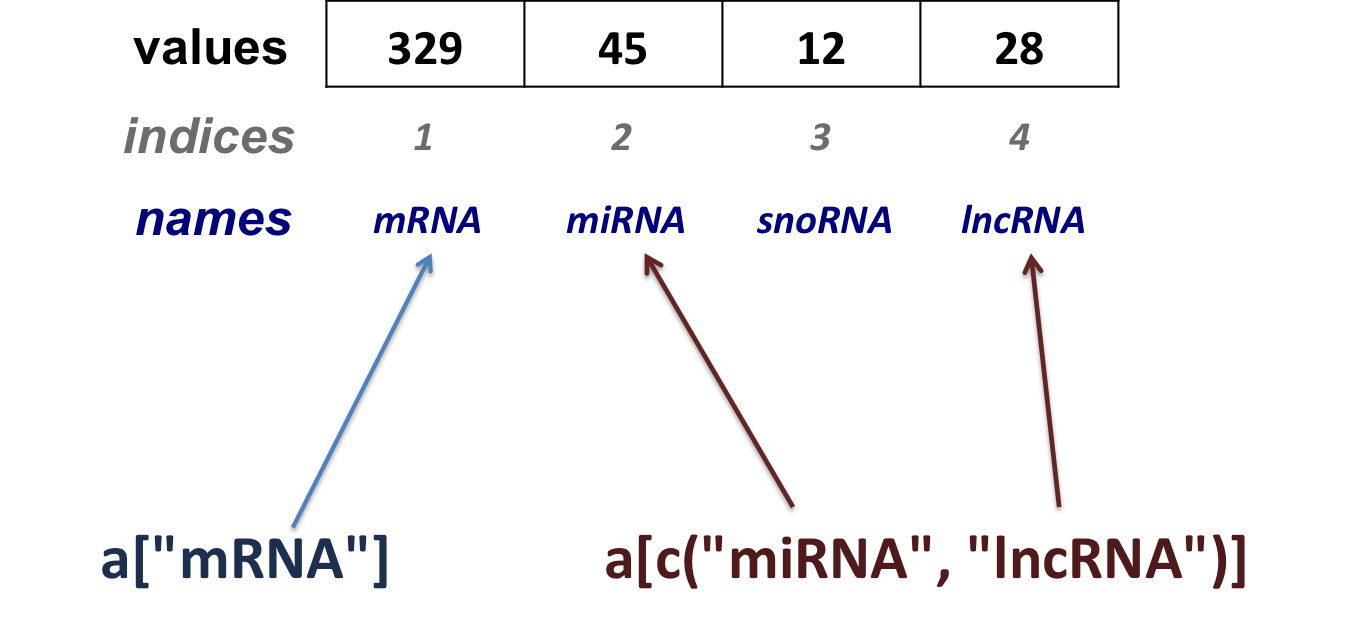``vector1["mRNA"]``
``````## mRNA
##  329``````
``vector1[c("miRNA", "lncRNA")]            ``
``````##  miRNA lncRNA
##     45     28``````
• Reassigning a vector’s element
``````# here we re-assign the 2nd element of vector1 with 31.
vector1 <- 31
vector1["miRNA"] <- 31``````
• Removing a vector’s element
``````# here we remove the 3rd element of vector1
# show in the console vector1 without its 3rd element:
vector1[-3]``````
``````##   mRNA  miRNA lncRNA
##    329     31     28``````
``````# reassign vector1 without its 3rd element
vector1 <- vector1[-3]``````
• Show versus change

x[-2]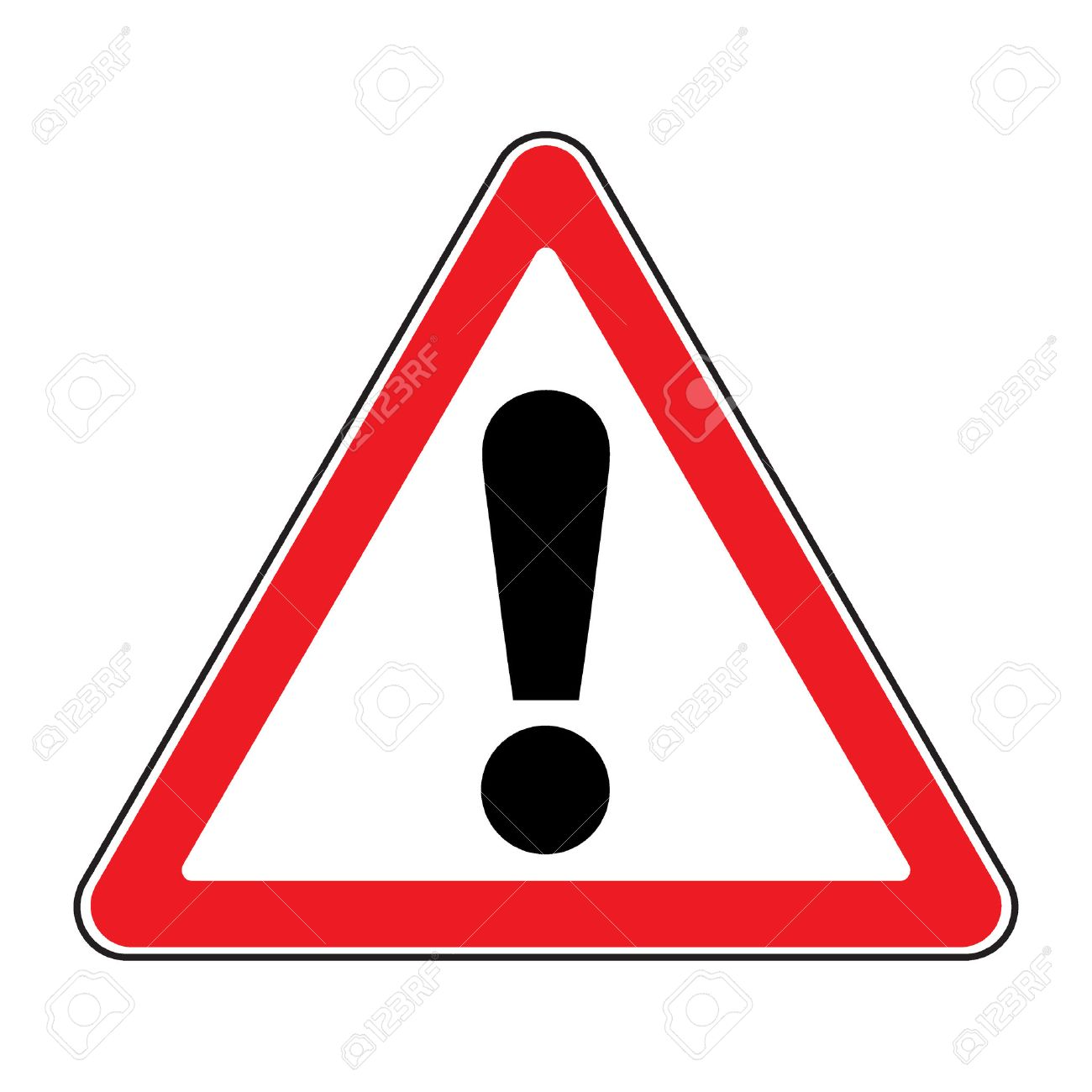x unchanged !

x <- x[-2]x reassigned !

### 9.1.3 Combining vectors

• You can combine 2 (or more) vectors into one. Here, we combine v1 and v2 to create a vector v3
``````v1 <- 2:5
v2 <- 4:6
v3 <- c(v1, v2)``````

The elements of v2 are added after the elements of v1

• Likewise, you can add elements at the end of a vector
``v3 <- c(v3, 19)``

### 9.1.4 Numeric vector manipulation

#### Logical operators

Operator Description
< less than
<= less than or equal to
> greater than
>= greater than or equal to
== exactly equal to
!= not equal to
!x not x
x | y x OR y
x & y x AND y
• Which elements of a are equal to 2?
``````a <- 1:5
a == 2``````
``##  FALSE  TRUE FALSE FALSE FALSE``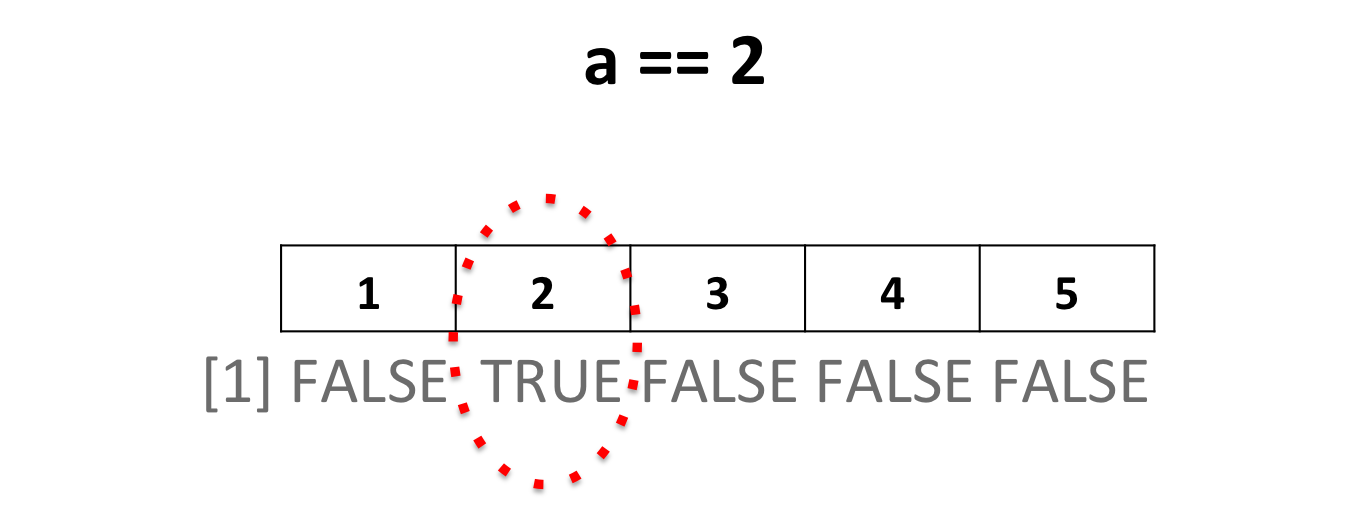• Which elements of a are superior to 2?
``````a <- 1:5
a > 2``````
``##  FALSE FALSE  TRUE  TRUE  TRUE``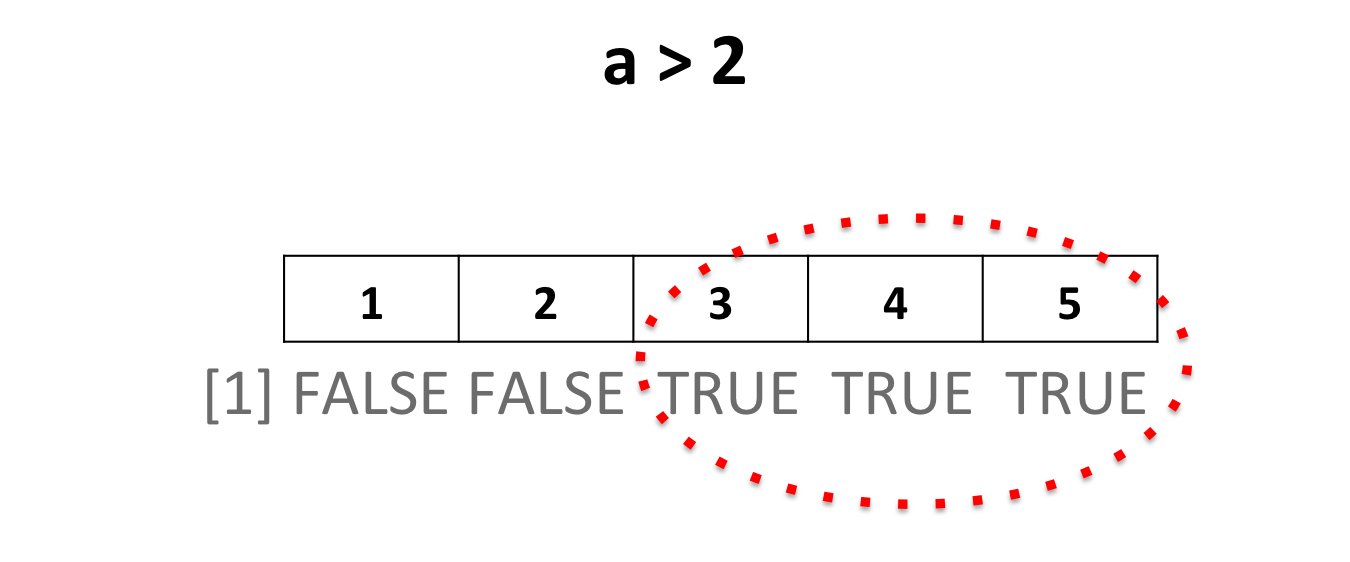• Extract elements of a vector that comply with a condition:
``````a <- 1:5
# extract the TRUE/FALSE vector
a >= 2``````
``##  FALSE  TRUE  TRUE  TRUE  TRUE``
``````# extract the actual sub-vector that complies the condition (TRUE values)
a[a >= 2]``````
``##  2 3 4 5``
``````# count how many elements comply the condition
length(a[a >= 2])``````
``##  4``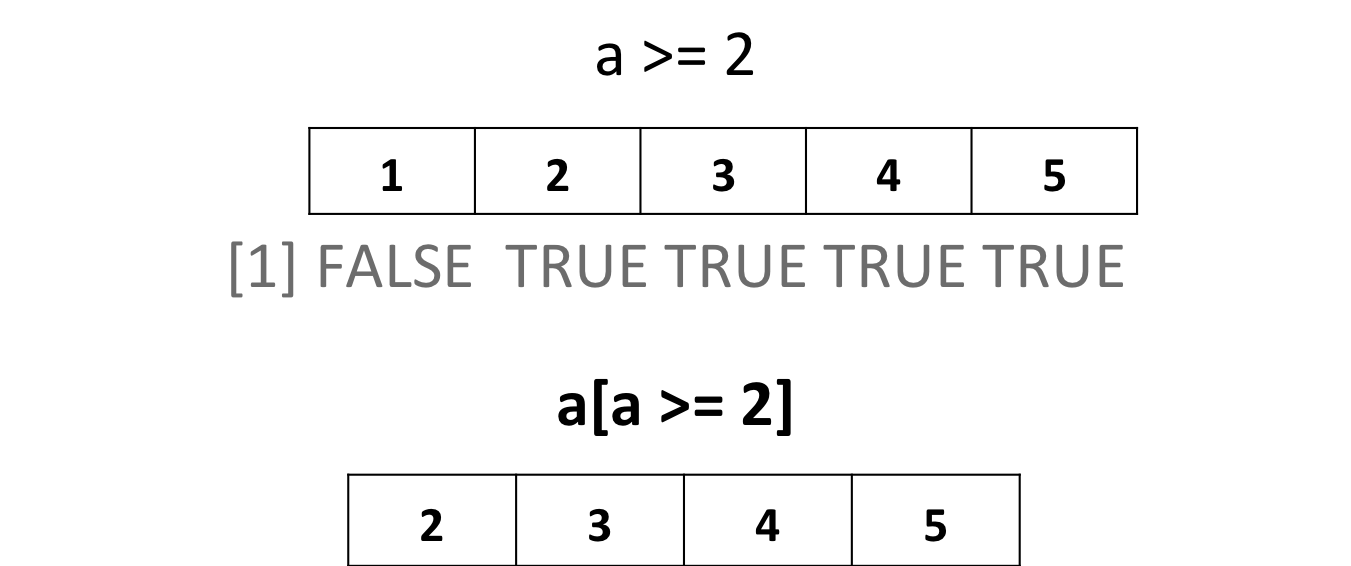HANDS-ON

Given the following vector containing a group of people’s ages:

``ages <- c(18, 32, 12, 34, 42, 32, 17, 56, 76, 10)``
1. How many people are over 18?
2. How many people are under 60?
3. How many people are between 25 and 45?

#### 9.1.4.1 Operations on vectors

• Adding 2 to a vector adds 2 to each element of the vector:
``````a <- 1:5
a + 2``````
``##  3 4 5 6 7``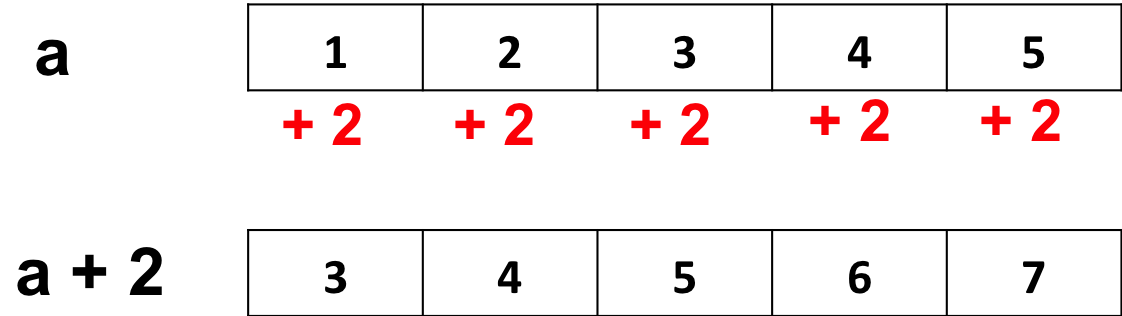Same goes for subtractions, multiplications and divisions…

• Multiplying a vector by another vector of equal length
``````a <- c(2, 4, 6)
b <- c(2, 3, 0)
a * b``````
``##   4 12  0``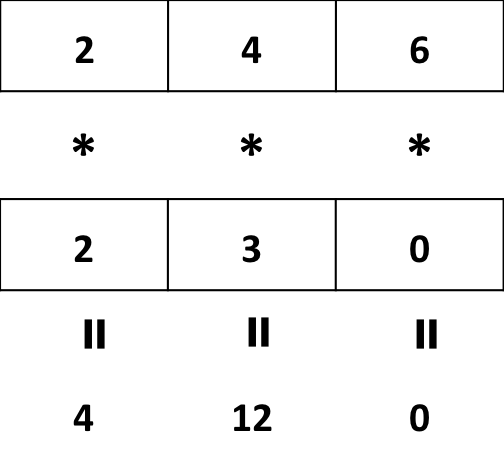• Multiplying a vector by another shorter vector
``````a <- c(2, 4, 6, 3, 1)
b <- c(2, 3, 0)
a * b``````
``## Warning in a * b: longer object length is not a multiple of shorter object length``
``##   4 12  0  6  3``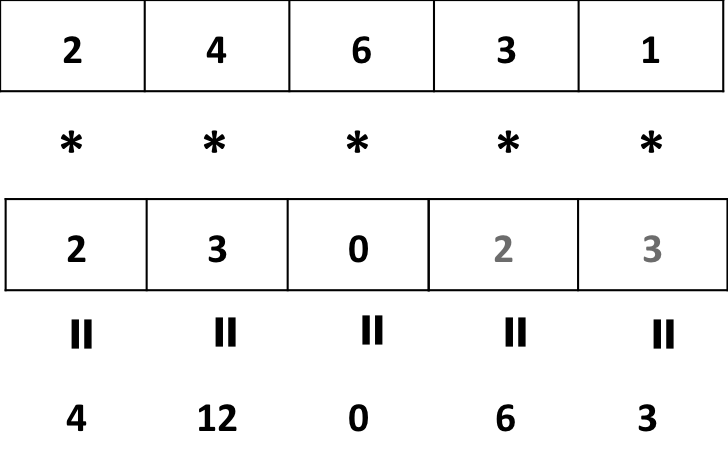Vector a is “recycled” !

• Summary statistics
Function Description
mean(x) mean / average
median(x) median
min(x) minimum
max(x) maximum
var(x) variance
summary(x) mean, median, min, max, quartiles
``````k <- c(1, 3, 12, 45, 3, 2)
summary(k)``````
``````##    Min. 1st Qu.  Median    Mean 3rd Qu.    Max.
##    1.00    2.25    3.00   11.00    9.75   45.00``````

HANDS-ON

Given our ages vector:

``ages <- c(18, 32, 12, 34, 42, 32, 17, 56, 76, 10)``
1. What is the average/mean age?
2. What is percentage of variation? It is defined as (standard deviation / mean) * 100.

#### 9.1.4.2 Comparing vectors

• The %in% operator

Which elements of a are also found in b ?

``````a <- 2:6
b <- 4:10
a %in% b``````
``##  FALSE FALSE  TRUE  TRUE  TRUE``

Yields a TRUE/FALSE vector of the size of a.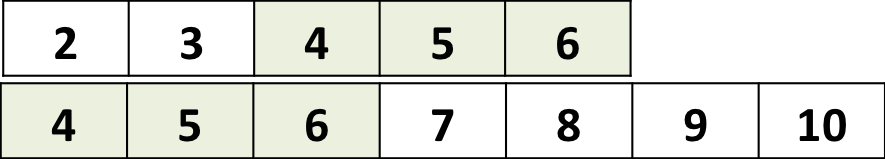Retrieve actual elements of a that are found in b:

``````a <- 2:6
b <- 4:10
a[a %in% b]``````
``##  4 5 6``
• Intersection:

Retrieve the intersection of 2 vectors:

``intersect(a, b)``
``##  4 5 6``
• Check if 2 vectors are exactly identical
``identical(a, b)``
``##  FALSE``

### 9.1.5 Character vector manipulation

Character vectors are manipulated similarly to numeric ones.

• The %in% operator:
``````k <- c("mRNA", "miRNA", "snoRNA", "RNA", "lincRNA")
p <- c("mRNA","lincRNA", "tRNA", "miRNA")
k %in% p``````
``##   TRUE  TRUE FALSE FALSE  TRUE``
``k[k %in% p]``
``##  "mRNA"    "miRNA"   "lincRNA"``
• Select elements from vector m that are not exon
``````m <- c("exon", "intron", "exon")
m != "exon"``````
``##  FALSE  TRUE FALSE``
``m[m != "exon"]``
``##  "intron"``